Java & Kotlin developer, blogger and tech enthusiast.

# Functions cheat sheetFunctional interface will have single abstract method and they provide target types for lambda expressions. There are many functional interfaces in Java and they all are available in java.util.function package. I tried to organize all of them with a logical diagram and table. Table contains interface name and abstract method. You can refer this article to get an idea of available functions and its variants.

### Consumer

Consumer takes input and always returns void. You can use this to perform some action with out returning anything.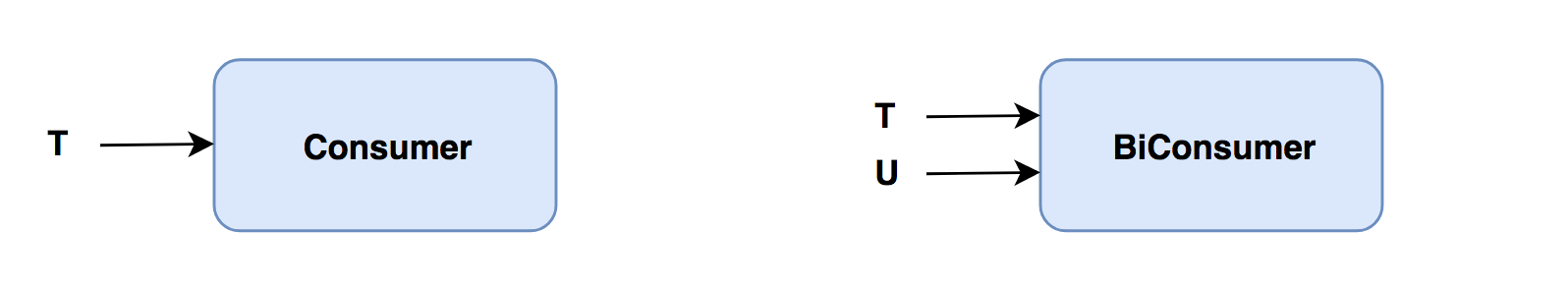Consumer void accept(T t) DoubleConsumer void accept(double value) IntConsumer void accept(int value) LongConsumer void accept(long value) BiConsumer void accept(T t, U t) ObjDoubleConsumer void accept(T t, double value) ObjIntConsumer void accept(T t, int value) ObjLongConsumer void accept(T t, long value)

### Function

Function takes input and produces different kind of output.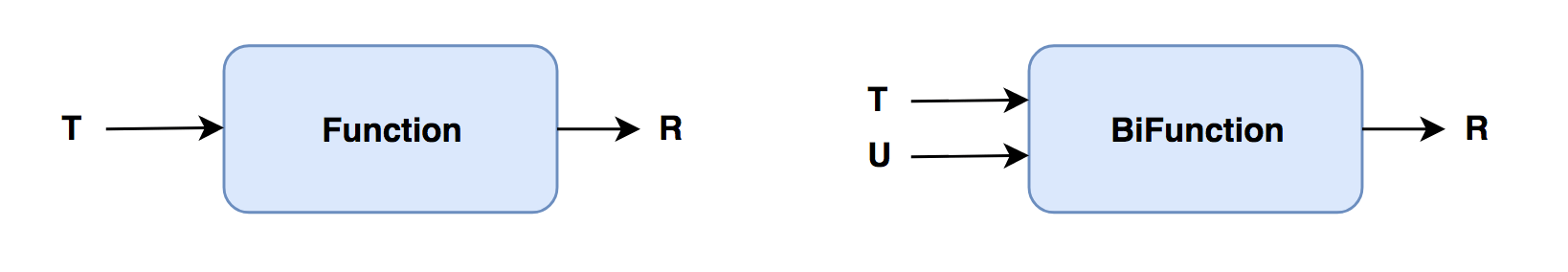Function R apply(T t) IntFunction R apply(int value) LongFunction R apply(long value) DoubleFunction R apply(double value) DoubleToIntFunction int applyAsInt(double value) DoubleToLongFunction long applyAsLong(double value) IntToDoubleFunction double applyAsDouble(int value) IntToLongFunction long applyAsLong(int value) LongToDoubleFunction double applyAsDouble(long value) LongToIntFunction int applyAsInt(long value) ToDoubleFunction double applyAsDouble(T value) ToIntFunction int applyAsInt(T value) ToLongFunction long applyAsLong(T value) BiFunction R apply(T t, U t) ToDoubleBiFunction double applyAsDouble(T t, U t) ToIntBiFunction int applyAsInt(T t, U t) ToLongBiFunction long applyAsLong(T t, U t)

### Operator

Operator is a special kind of function where it always accepts and produces same type.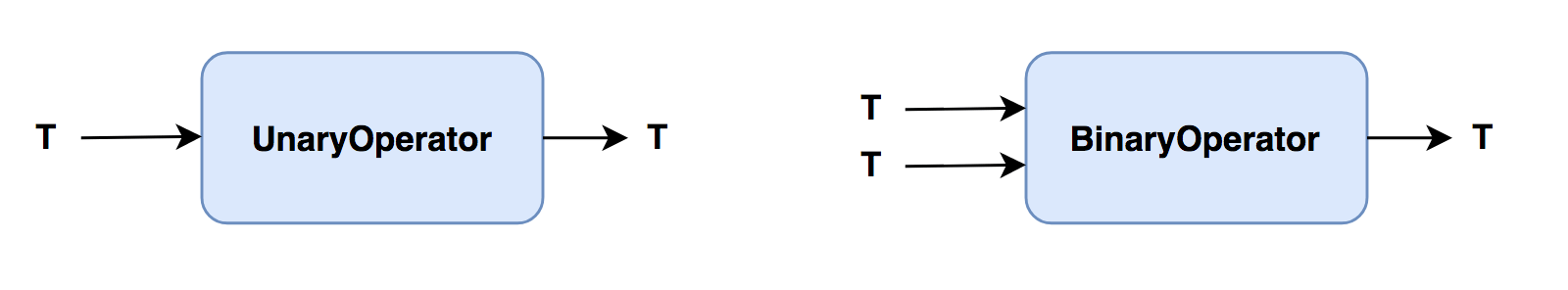UnaryOperator T apply(T t) DoubleUnaryOperator double applyAsDouble(double operand) IntUnaryOperator int applyAsInt(int operand) LongUnaryOperator long applyAsLong(long operand) BinaryOperator R apply(T t, U t) DoubleBinaryOperator double applyAsDouble(double left, double right) IntBinaryOperator int applyAsInt(int left, int right) LongBinaryOperator long applyAsLong(long left, long right)

### Predicate

Predicate always returns a boolean so you can this in conditional evaluations.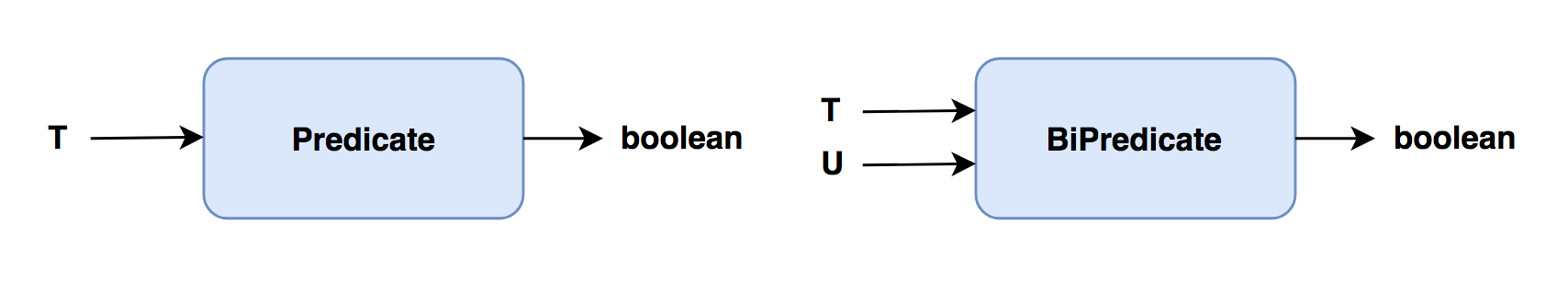Predicate boolean test(T t) DoublePredicate boolean test(double value) IntPredicate boolean test(int t) LongPredicate boolean test(long t) BiPredicate boolean test(T t, U u)

### Supplier

Supplier always returns a value. This can be used to generate random values or data from some source.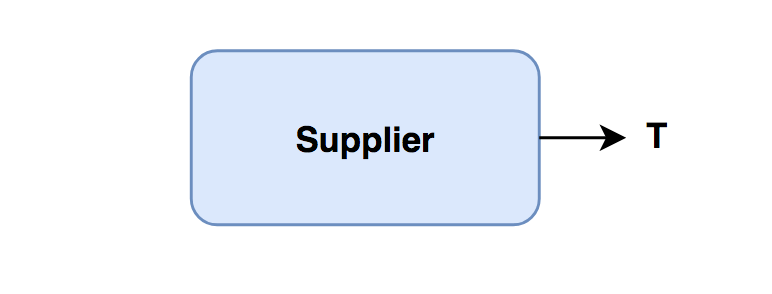Supplier T get() BooleanSupplier boolean getAsBoolean() DoubleSupplier double getAsDouble() IntSupplier int getAsInt() LongSupplier long getAsLong()